### Home > PC > Chapter 8 > Lesson 8.1.3 > Problem8-44

8-44.
1. Let k(x) =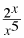and m(x) = log(k(x)). Homework Help ✎

1. Find m(x) by substituting and using the properties of logarithms to simplify the expression.

2. Find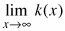and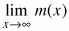.

3. Check your results by graphing or by using the [TABLE] command on your calculator, whichever you prefer.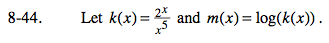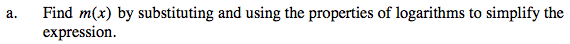$m(x)=\text{log}\left( \frac{2^x}{x^5} \right)$

$=\text{log}(2^x)-\text{log}(x^5)$

$=x\text{log}(2)-5\text{log}(x)$

$\approx0.301x-5\text{log}x$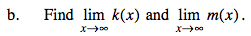For k(x), he dominant term isin the numerator.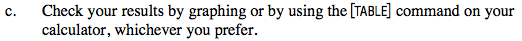Use the eTool below to solve this problem.
Click the link at right for the full version of the eTool: PCT 8-44 HW eTool